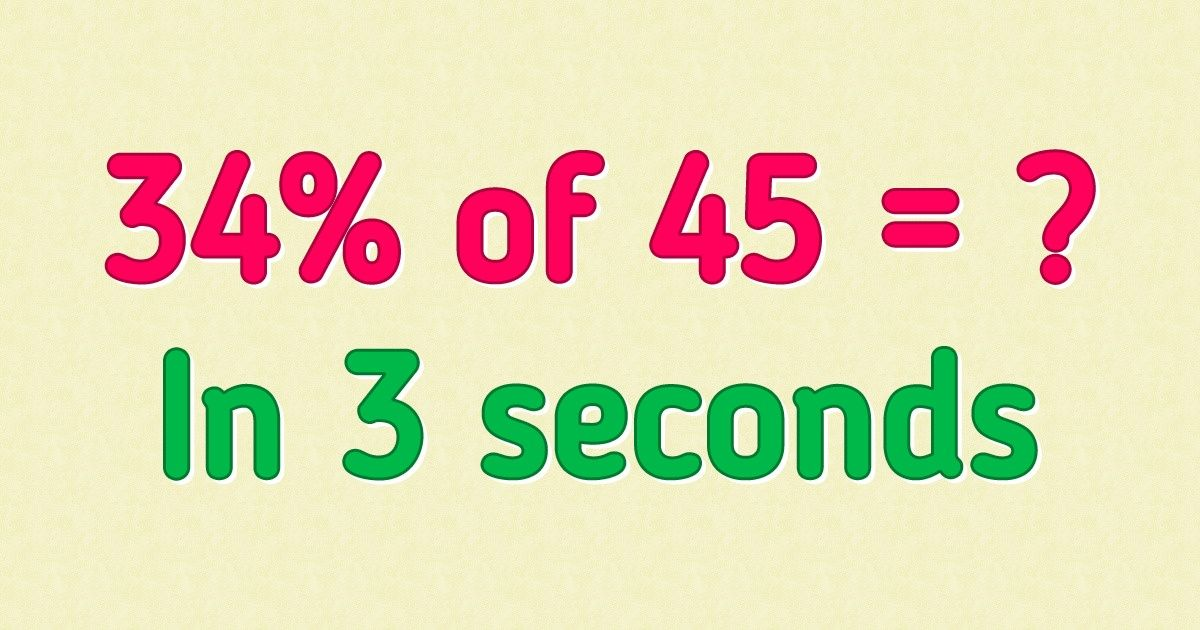# How to Calculate Percentages

We were taught how to calculate percentages at school, but by the time we hit adulthood, many of us lost this important skill. Whether you’re trying to calculate a discount at the store or are thinking about what amount of money you should save every month to go on your dream vacation, you need to know the correct math formula.

5-Minute Crafts will show you several ways to calculate percentages that can be used in different situations.

### Basic rule

If you need to calculate the percentage of a number, you should use the formula P% * X = Y where:

• P% is the percentage.
• X is the number itself.
• Y is the final answer.

For example, you may need to figure out how much money you’ll save if you buy a smartphone that costs \$250 with a 20% discount.

1. In this case, the equation will be: 20% * 250 = Y
2. To do the math, you should convert 20% to a decimal. Just divide the number by 100 and remove the percentage sign. You should get: 20/100 = 0.2
3. Change the equation: 0.2 * 250 = Y
4. Do the calculations: 0.2 * 250 = 50. The answer is Y = 50.

You’ll save \$50 if you get a smartphone with a 20% discount.

### An alternative way

There’s another way to calculate percentages that may come in handy when you don’t have a calculator on hand and the equation turns out to be pretty complex.

Let’s assume you still need to figure out what 20% of 250 is.

1. To do it, you can multiply the first numbers without zeros: 2 * 25 = 50.
2. Then, you’ll need to figure out what to do with the zeros you didn’t take into consideration when multiplying the numbers. So the answer you get could be 50, 0.5, or 500.
3. The last 2 numbers are either too small or too big in comparison to 250. It’s impossible that 0.5 and 200 constitute 20% of 250. So 50 is the correct answer.

If you have to work with more complex numbers, you can modify this approach a bit. Let’s say you need to calculate 34% of 45.

1. Break 34% into 30% + 4%.
2. This way, the equation will be (30% + 4%) * 45.
3. Do the math: (30% + 4%) * 45 = 13.5 + 1.8 = 15.3.

Another example — let’s calculate 40% of 154.

2. This way, the equation will be 40% * (150 +4).
3. Do the math: 40% * (150 + 4) = 60 + 1.6 = 61.6.

### 2. Calculating what percent a certain number is of a bigger number

If you need to calculate what percent a certain number makes up of a bigger number, you should use the formula Y/X = P%.

For example, let’s assume that you spent \$20 on new shoes from the \$80 gift you got for your birthday, and now you want to calculate what percentage of your gift you’ve already spent.

1. In this case, Y = 20 and X = 80.
2. The equation will be 20/80 = P%.
3. Do the calculations: 20/80 = 0.25.
4. Then, you need to convert the result you got in decimal form into the percentages. To do it, you should multiply the number you got by 100.
5. The equation will be: 0.25 * 100 = 25%. The answer is 25%.

### 3. Figuring out the whole number if you already know the percentage and how much it is

If you need to calculate the whole number when you already know the percentage and how much it constitutes, you should use the formula Y/P% = X.

For example, you may see an ad that states you’ll save \$40 or 20% of the total cost of the weekend trip if you book it right away. You’re curious about the total cost of the trip but the ad mentions nothing about it. So you decide to calculate it yourself.

1. In this case, Y = 40, P% = 20%, and X is unknown.
2. The equation will be 40/20% = X.
3. Turn 20% into a decimal: 20/100 = 0.2.
4. The equation will be 40/0.2 = X.
5. Do the math: 40/0.2 = 200. The answer is 200.

So the total cost of the trip is \$200.

5-Minute Crafts/Tricks/How to Calculate Percentages